Maths-
General
Easy

Question

# How can you use polynomial identities to factor polynomials and simplify numerical expressions ?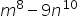Hint:

## The correct answer is: m8 - 9n10 = (m4 - 3n5)(m4 + 3n5)

### Step 1 of 2:The given expression is. It can be written as,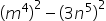. Here,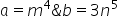. Thus, we can apply the identity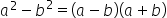to simplify the expression.Step 2 of 2:Simplifying the expression, we get: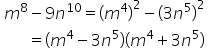Thus, the simplified expression is: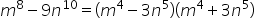We use polynomial identities to reduce the time and space while solving polynomial expressions and equations.#### With Turito Foundation.#### Get an Expert Advice From Turito.